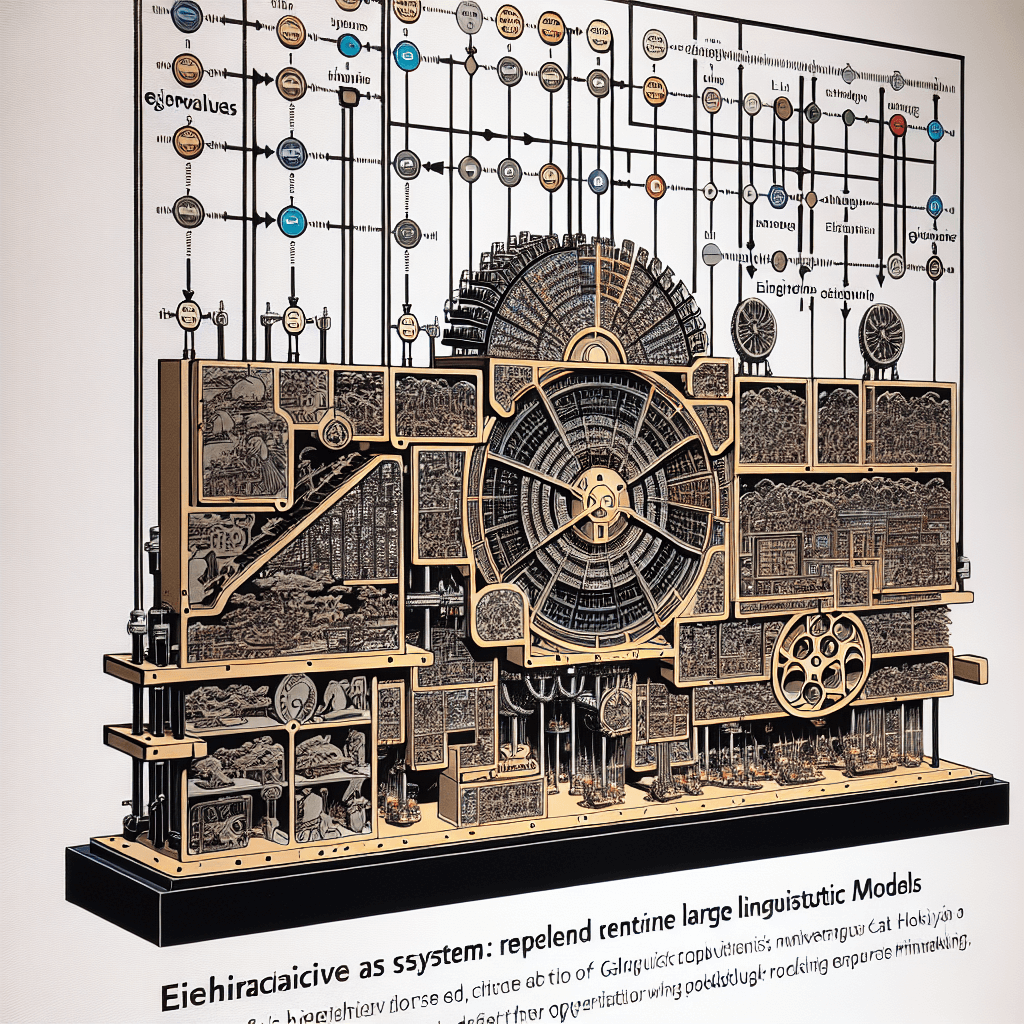# Week 4: Eigenvalues - Unveiling the Hidden Power of Matrices

syndu | Oct. 4, 2023, 4:52 p.m.# Week 4: Eigenvalues - Unveiling the Hidden Power of Matrices

Hello learners! Welcome to Week 4 of our journey into the fascinating world of linear algebra. This week, we're going to delve into a key concept that's as intriguing as it is important: Eigenvalues.

## What are Eigenvalues?

Eigenvalues are a special set of scalars associated with a linear system of equations (i.e., a matrix equation) that are sometimes also known as characteristic roots, characteristic values, proper values, or latent roots.

The term "eigenvalue" comes from the German word "eigen," which means "own" or "particular to." So, an eigenvalue is a value that is particular to a given matrix.

## Why are Eigenvalues Important?

Eigenvalues play a crucial role in many areas of mathematics and its applications, including the stability analysis in differential equations, the analysis of networks, and even Google's PageRank algorithm. They are also used in many areas of science and engineering, such as vibration analysis, atomic research, studying population growth, and so on.

## How to Find Eigenvalues?

To find the eigenvalues of a matrix, we need to solve the characteristic equation, which is given by `det(A - λI) = 0`, where:

• A is the matrix,
• λ represents the eigenvalues,
• I is the identity matrix of the same size as A,
• det denotes the determinant of a matrix.

The roots of this equation are the eigenvalues of the matrix A.

Let's illustrate this with an example:

Consider a 2x2 matrix A:

``````
A = [4 1]
[2 3]
``````

The characteristic equation is given by `det(A - λI) = 0`, which simplifies to `(4-λ)(3-λ) - (2*1) = 0`. This further simplifies to `λ^2 - 7λ + 10 = 0`. The roots of this equation, λ = 2 and λ = 5, are the eigenvalues of the matrix A.

Remember: Eigenvalues are a fundamental concept in linear algebra with wide-ranging applications. Understanding them will open up new dimensions in your mathematical journey.

## Wrapping Up

So, keep practicing, keep exploring, and remember that every matrix holds its own unique 'eigen' secrets waiting to be discovered!

Next week, we'll build on this foundation and explore the concept of eigenvectors. Stay tuned!

Happy learning!

##### Lilith's Grimoire

The app you are using right now is Lilith's Grimoire

The Grimoire is full of Source Code. It is alive, and running, and you can explore the way it is built

The first lesson uncovers all of it.#### Understanding Matrix Determinants, Cofactor Expansion, and Systems of Linear Equations#### Understanding and Calculating Determinants#### The Role of Eigenvalues and Eigenvectors in Large Language Models#### Eigenvalues and Eigenvectors: Their Relevance to Large Language Models#### Linear Algebra in Neural Networks: A Comprehensive Introduction#### Basics of Linear Algebra: Vectors, Matrices, and Operations#### Empowering Gifted Learners with Next-Generation Large Language Models